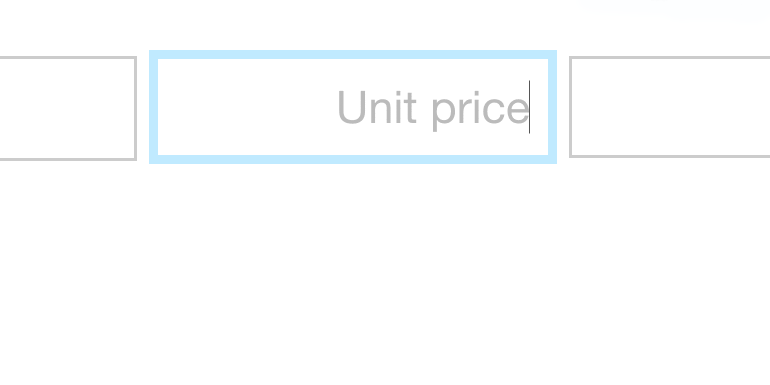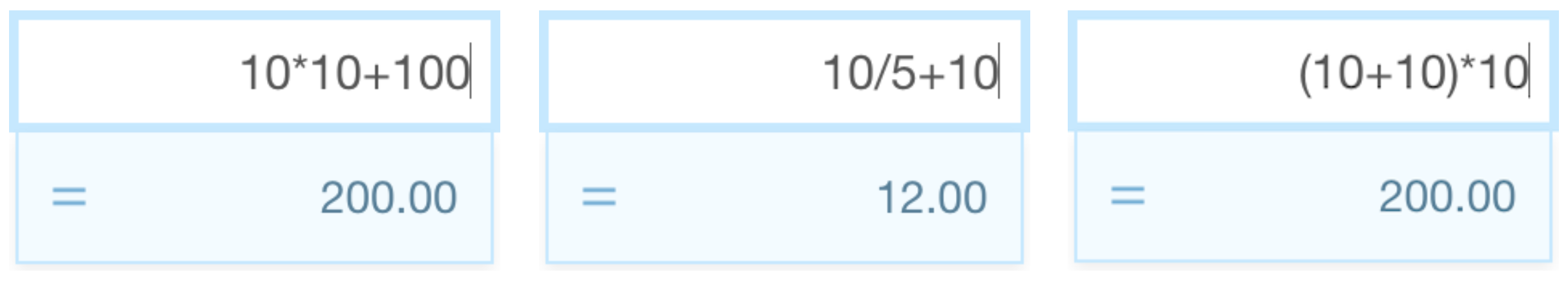# Make calculations inside Quotient

You might find that there are times when you need to perform simple calculations for your Quotes. For example, you need to calculate the area of a room, or the total number of widgets being supplied from a whole pile of boxes.

With Quotient you can make simple calculations without leaving the app. In fact, any number field can be used to perform basic math equations.Here are some examples of the kinds of calculations you can perform:Note: The equations don't save/stick once the calculation has been completed – only the resulting answer is saved.